# Multilevel Modelling and PPC Bids

This document is hopefully a better presentation of what I tried to talk about at MeasureCamp 7.

Last updated on Wednesday 09 January 2019

This is a fairly mathematical topic (or it is when you approach it like I do) but I think there should be something useful here even for those who don’t care for maths.

## A Brief Overview of PPC Bid Management

In very broad terms bid management is quite simple. The detail is complicated but the high level view is not. A bid management system must do the following for each thing that is bid upon (normally a keyword):

1. Estimate the value of a click
2. Apply some business rules to this to get a target cost per click
3. Set a bid to get the actual cost per click as close to the target as possible

This document covers a small bit of step 1. I won’t really talk about incrementality or volatility, both of which can be far more important in some circumstances.

Another simplification I will make it to ignore the wild variety of different values that a conversion can have and turn it into a binary event. If the user converts then their click has value 1. If they didn’t then their click has value 0.

Obviously, this is not how things work but looking at things this way makes the maths way easier to start with and layering in a more complicated value model on top of these results isn’t that hard. If you are in a position to apply the rest of what I’m saying here then this small obstacle should be solvable for you.

## Binomial and Beta Distributions

With the simplification to a binary outcome (a conversion is always worth 1. A none conversion is worth 0) our value per click calculation comes down to figuring out our best estimate of the conversion rate.

I’m going to assume that all users clicking though on a given keyword are identical and then they convert at random. The chance that a user converts is the true conversion rate of the keyword.

This assumption sounds a bit stupid, but it is one that everyone makes even if they don’t realise it. And it gives us a big toolbox of statistical tools that we can start to use.

The true conversion rate is the underlying probability of a user converting which is quite different to the observed conversion rate; this is the number of conversions divided by the number of clicks.

Here is some code to show the difference between true and observed conversion rates:

I won’t be showing all the code for everything in this document. This code is here to show the sampling from a binomial distribution

    library(scales) # for percent formatting

keywords <- c("dog skateboards",
"xtreme pooch helmet")
true_conversion_rate <- 0.05 #same for all keywords
number_of_clicks <- c(100,50,10)

# rbinom samples from a binomial distribution
# sapply is kind of an R version of "map"
number_of_conversions <- sapply(number_of_clicks,
function(x) {
rbinom(1, x, true_conversion_rate)
}
)

observed_conversion_rate <- number_of_conversions/number_of_clicks

keyworddf <- data.frame(Keyword=keywords,
Clicks=number_of_clicks,
Conversions=number_of_conversions,
truerate=percent(rep(true_conversion_rate,3)),
observedrate=percent(observed_conversion_rate)
)
names(keyworddf) <- "True Conv Rate"
names(keyworddf) <- "Observed Conv Rate"
kable(keyworddf)
Keyword Clicks Conversions True Conv Rate Observed Conv Rate
dog skateboards 100 3 5% 3%
rollarblades for dogs 50 4 5% 8%
xtreme pooch helmet 10 0 5% 0%

In this example every keyword has the same true conversion rate but, as you can see, the observed conversion rates are quite different.

The process of estimating the true value of a binomial parameter from noisy data is the same process used when trying to estimate the chance that a biased coin will land heads. Wikipedia has a page on this which goes over the maths in a bit more detail.

If you follow the maths on wikipedia, or if you take my word for it, you’ll see that (under some assumptions that we’ll get to later) our posterior estimate for a conversion rate is

β(conversions + 1, clicks − conversions + 1)

We’re already somewhere quite confusing here in two ways:

1. We’ve got a distribution rather than a point estimate
2. WTH does β mean?

### Point Estimates vs Distributions

A point estimate is where you say something like “the average height is 1.843m”. A distribution is where you say something like "height is normally distributed with mean 1.8m and variance 0.08.

    library(ggplot2)
xs <- seq(0.8,2.8,by=0.01)
ys <- dnorm(xs, mean=1.8, sd=sqrt(0.08))
df <- data.frame(Height=xs,density=ys)
ggplot(df,aes(x=Height,y=density)) +
geom_line(color="green") +
theme_minimal() +
ylab(NULL) +
scale_y_continuous(labels=NULL) +
geom_vline(xintercept=1.8,color="blue") +
annotate("text",label="Point estimate", x=1.92, y=0.1, color="blue") +
annotate("text",label="Distribution", x=1, y=0.1, color="green")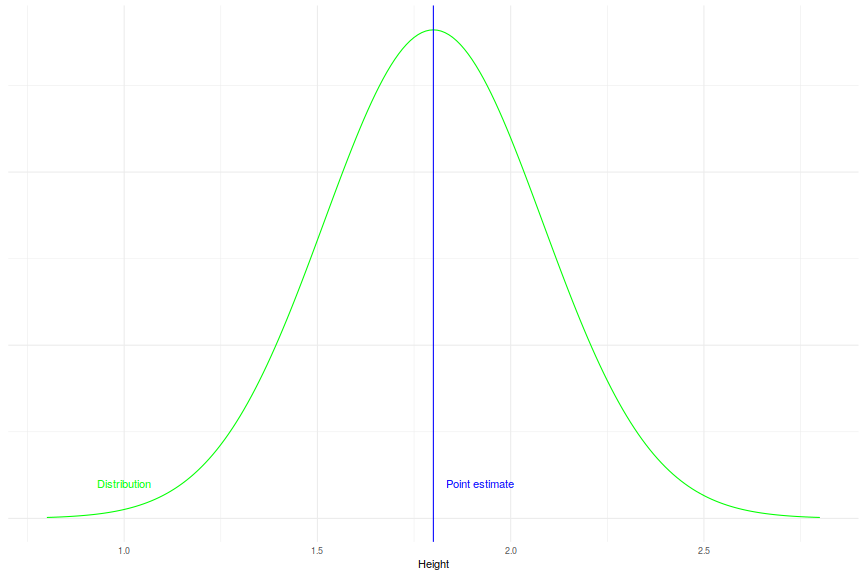Using the distribution allows you to answer questions that you can’t answer with a point estimate. And it is easy to get a point estimate from a distribution which isn’t the case in the other direction.

For example, if you make furniture there may be a business requirement that is fit everyone except the tallest 5% of people. Knowing the point estimate for average height (1.8m) doesn’t help you do this but if you have an estimate of the distribution you know that you need to fit everyone less than 2.2652349m. [made up numbers - I’m fairly sure way more than 95% of people are smaller than that!]

This is an important idea when setting bids because it allows you to choose the “aggressiveness” of your strategy. Because conversion is a random process you never know for sure that you will make money - it could be that no one ever buys anything from you.

With knowledge of the distributions you can say “I want to be 99% sure I will make money” and set a relatively low bid compared to someone who says “I want to be 50% sure I will make money”. I’ll talk a bit more about this after I’ve introduced the Beta distribution in the next section.

### The Beta distribution

Most people have heard of the normal distribution which is defined in terms of the mean and the variance of the data. The mean and variance are known as sufficiant statistics for this distribution.

The beta distribution is a different distribution which can be defined in terms of the number of successes and the number of failures of a binomial trial. (Remember that in our assumption, a conversion is a success and a click that doesn’t convert is a failure).

Here are some plots of how the beta distribution looks for different combinations of clicks and conversions:

 betadata <- function(clicks,conversions) {
xs <- seq(0,1,by=0.001)
ys <- dbeta(xs,conversions+1,clicks+1-conversions)
data.frame(xs=xs,d=ys)
}

makeplot <- function(clicks,conversions) {
maxdent <- max(df$d) ggplot(df,aes(x=xs,y=d)) + geom_line() + theme_minimal() + xlab("Conv rate") + ylab(NULL) + scale_y_continuous(labels=NULL) + expand_limits(y=0) + annotate("text", label=paste("Clicks: ",clicks,"\nConversions: ", conversions,sep=" "), x=0.7, y=maxdent*0.7) } multiplot(makeplot(5,0), makeplot(50,10), makeplot(1000,200), makeplot(5,1), makeplot(500,100), makeplot(5000,1000), cols=2)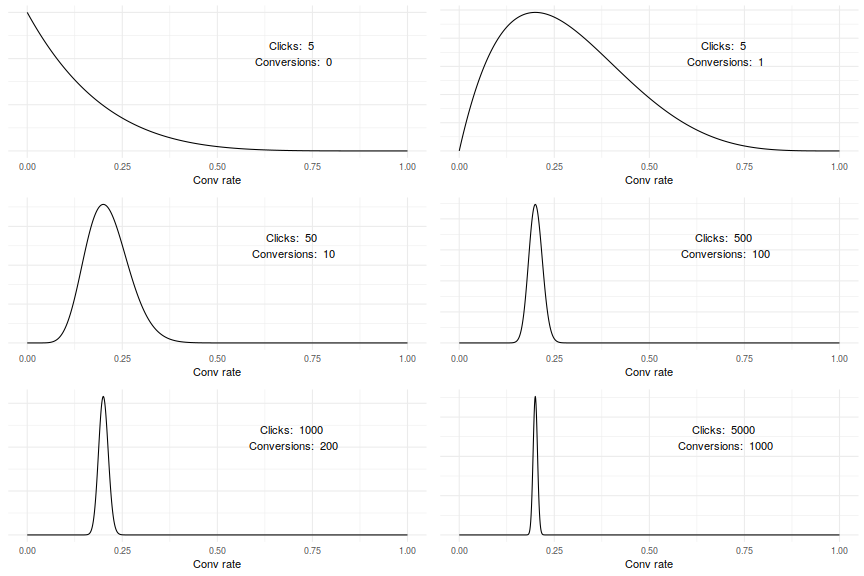As you can see, as more data comes in the distribution “narrows” around a true value. This is what we’d hope to see! #### Bidding Example So suppose we have a target cost per conversion of £10 and we want to be 80% sure we’ll make money off a keyword that has 50 clicks and 10 conversions. If we only use the point estimate then we’d say something like “the conversion rate is 20% - we can pay £2 per click” but this ignores the 80% criteria that we want to include in our estimate. With the distribution approach, first ask “what value are we 80% sure the conversion rate is higher than?” - here this is 0.1630874 so we should bid less than what the point estimate suggests.  df <- betadata(50,10) df_high <- df[ df$xs >= qbeta(0.2,10+1,50-10+1),]
ggplot(df,aes(x=xs,y=d)) +
geom_line(color="blue") +
geom_ribbon(data=df_high, aes(x=xs, ymax=d), fill="blue", ymin=0) +
theme_minimal() +
xlab("Conv rate") +
ylab(NULL) +
scale_y_continuous(labels=NULL) +
annotate("text", label="There is an 80% chance the\ntrue conversion rate is in\nthe shaded area.",x=0.8,y=6, color="blue")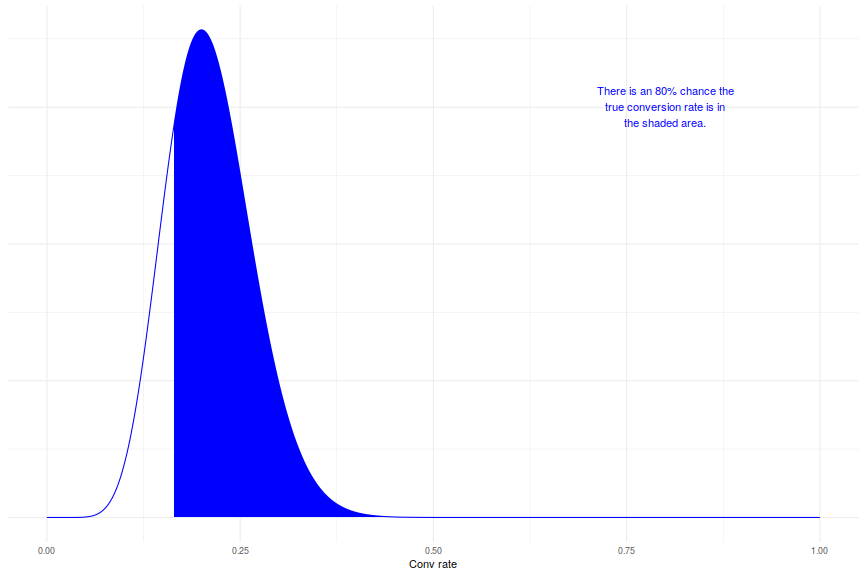## Prior information

This is all very well until you look at a beta distribution for a keyword with no data:

    df <- betadata(0,0)
ggplot(df,aes(x=xs,y=d)) +
geom_line() +
theme_minimal() +
xlab("Conv rate") +
scale_y_continuous(labels=NULL,limits=c(0,2)) +
ylab(NULL) +
annotate("text", label=paste("Clicks: ",0,"\nConversions: ", 0,sep=" "), x=0.7, y=1.5)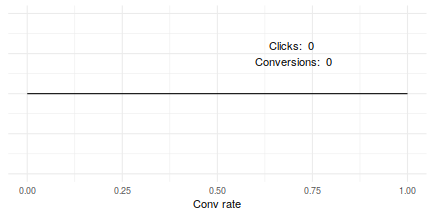The chart shows that for a keyword with zero clicks and zero conversions it is just as likely that the true conversion rate is 100% as the true conversion rate is 10% or 20% or π%.

Is this true?

I don’t think this is true. I think it is way less likely that a keyword has a 100% conversion rate than that it has a 10% or 20% conversion rate. There are three things that could be going on here:

1. My intuition doesn’t fit reality
2. The model doesn’t fit reality
3. Both of the above

Right now I’m going to go with “I am right and the model is wrong” and try to come up with a better model that doesn’t have this problem. If you want to argue with me that a 100% conversion rate is equally likely then drop me a line.

Why do I believe that a 100% conversion rate is unlikely even when I have no data?

Because across all the accounts I’ve ever managed I’ve never seen anything like this. Why should this one new keyword be so different to all the other ones I’ve ever seen?

My brain is intuitively combining what it has seen in the past (lots) with the new information we have on this keyword (not much). The model we were using above does not take this into account so it approaches each new keyword with a completely naive background. We want a model with some way of incorporating this extra information.

I’m not sure it is realistic to try and incorporate data from every keyword I’ve ever seen into the estimate of the conversion rate for a new keyword. But it is possible to incorporate data from other keywords in the account.

Some of you might be thinking “why bother with all this - just run the keyword for a bit and get some data”.

The rest of this document is probably not for you.

We want a model that somehow includes the intuition “This keyword is probably quite like the other keywords in the account”.

A solution to this is quite simple if go back to just using point estimates. Let convrobserved be the observed conversion rate for a keyword and let convraccount be the conversion rate for the whole account. Then we can use the following formula to make an estimate of the true conversion rate for a keyword:

estimate = λconvrobserved + (1 − λ)convraccount

λ is a number between zero and one. When λ = 1 we use just the observed conversion rate (ignoring the account information) and when λ = 0 we use entirely the account average conversion rate as our estimate (ignoring any keyword specific information). Values between zero and one blend the two together into a hybrid estimate.

An easy way to get started with this is to pick a high value of λ (e.g. 0.95 or 0.99) and use this across all keywords. A more advanced method is to scale the value of λ based on the number of clicks. Where a keyword has a lot of data use a larger value of λ and where there is not much data use a smaller value to give more weight to the account average.

Here is some R code which does this for the example data from earlier and plots the results:

Code presented to show just how simple this is. You could even do it in Excel

   keyworddf[,4] <- NULL
keyworddf$"Observed Conv Rate" <- as.numeric(gsub("%","",keyworddf$"Observed Conv Rate"))/100
kable(keyworddf)
Keyword Clicks Conversions Observed Conv Rate
dog skateboards 100 3 0.03
rollarblades for dogs 50 4 0.08
xtreme pooch helmet 10 0 0.00
    account_convr <- 0.02 # account conversion rate

generateLambda <- function(clicks) {
ifelse(clicks > 30, 0.90, 0.3)
}

keyworddf$lambda <- generateLambda(keyworddf$Clicks)

keyworddf$estimatedconvr <- keyworddf$lambda * keyworddf$"Observed Conv Rate" + (1-keyworddf$lambda) * account_convr
kable(keyworddf)
Keyword Clicks Conversions Observed Conv Rate lambda estimatedconvr
dog skateboards 100 3 0.03 0.9 0.029
rollarblades for dogs 50 4 0.08 0.9 0.074
xtreme pooch helmet 10 0 0.00 0.3 0.014
    keyworddf <- keyworddf[-2,]
ggplot(kwd1,aes(x=xs,y=d)) +
geom_line(color="blue", alpha=0.3) +
geom_line(data=kwd2,aes(x=xs,y=d),color="green", alpha=0.3) +
theme_minimal() +
xlab("Conv rate") +
scale_y_continuous(labels=NULL) +
ylab(NULL) +
xlim(0,0.1) +
geom_vline(xintercept=0.05,color="blue") +
geom_vline(xintercept=0.047,color="blue") +
geom_vline(xintercept=0, color="green") +
geom_vline(xintercept=0.014, color="green") +
annotate("text",label="Estimate for keyword with more data\nis dragged a small amount towards\nthe account average",
color="blue",
x=0.076,
y=5,
size=4.5) +
annotate("text",color="green",label="Estimate for keyword with\nless data is dragged\na larger amount towards\nthe account average",
x=0.029,
y=5,
size=4.5
)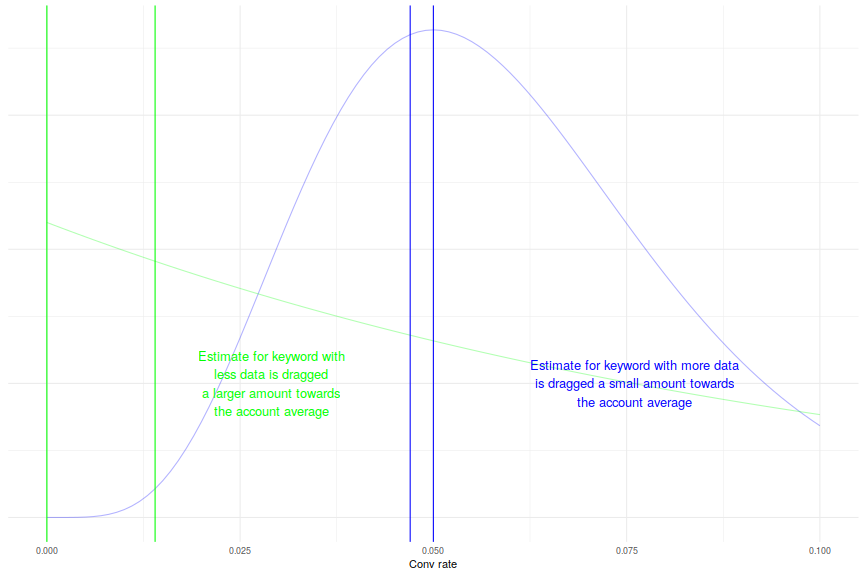This is great(ish). But it only gives us a point estimate and we’ve got all these manually specified λs all over the place. It is possible to make data driven choices for λ but this still doesn’t get us any insight into the distribution.

We can do better - we can get an estimate of the distribution and we can do it in a way that better represents our uncertainty about how much the overall account average should influence our estimate of the keyword conversion rate.

For our basic model we are trying to estimate a single parameter (the conversion rate) for each keyword in the account. When we have no data for a keyword there is a hidden assumption that any value for the conversion rate is as likely as any other - I think this assumption is false and I want to make a new model with different assumptions.

The conjugate prior for a binomial distribution is a beta distribution which means it is nice and easy for us to encode our prior information as a beta distribution.

This means that if we can start with a beta distribution and then update it using data from a binomial distribution in a simple way.

Hopefully this will become clearer with an example:

### Example

I need to think of a way of turning my intuitive thoughts about what the conversion rate for a keyword will be into a beta distribution. For this example, I expect the conversion rate to be about 15% but I wouldn’t be that surprised if it was a bit more or a bit less than that.

Here is a chart showing my “belief” that the conversion rate is a particular value before I’ve seen any data:

    df <- betadata(100,15)
ggplot(df,aes(x=xs,y=d)) +
geom_line() +
theme_minimal() +
xlab("Conv rate") +
scale_y_continuous(labels=NULL) +
ylab(NULL)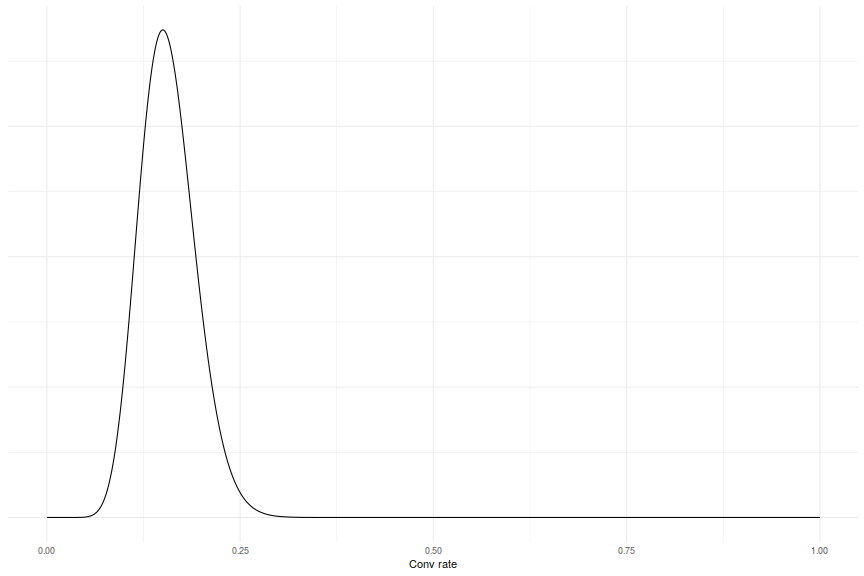This plot is just the probability density function for a beta distribution for a keyword with 100 clicks 15 conversions.

Incorporating this prior information into our modelling is easy:

    # the function betadata generates points from
# the beta distribution that arises from the
# given number of clicks and conversions

# without prior information

# with prior information
density <- betadata(clicks+100,conversions+15)

So it is as if every keyword starts off with 100 clicks and 15 conversions.

If the true conversion rate is not 15% but 25%, we can see how our distribution updates as more data is gathered. The charts on the left are our new estimates with the prior information. The charts on the right are the naive estimates with a uniform prior:

I wish this was an animated gif. If anyone can figure out how to embed these in a Knitr document please let me know

    makeplotPrior <- function(clicks,conversions) {
maxdent <- max(df$d) ggplot(df,aes(x=xs,y=d)) + geom_line() + theme_minimal() + xlab("Conv rate") + ylab(NULL) + scale_y_continuous(labels=NULL) + expand_limits(y=0) + annotate("text", label=paste("Clicks: ",clicks,"\nConversions: ", conversions,sep=" "), x=0.7, y=maxdent*0.7) } multiplot(makeplotPrior(0,0), makeplotPrior(10,1), makeplotPrior(20,7), makeplotPrior(40,9), makeplotPrior(80,19), makeplotPrior(120,27), makeplotPrior(200,59), makeplotPrior(500,118), makeplotPrior(1000,258), makeplot(0,0), makeplot(10,1), makeplot(20,7), makeplot(40,9), makeplot(80,19), makeplot(120,27), makeplot(200,59), makeplot(500,118), makeplot(1000,258), cols=2)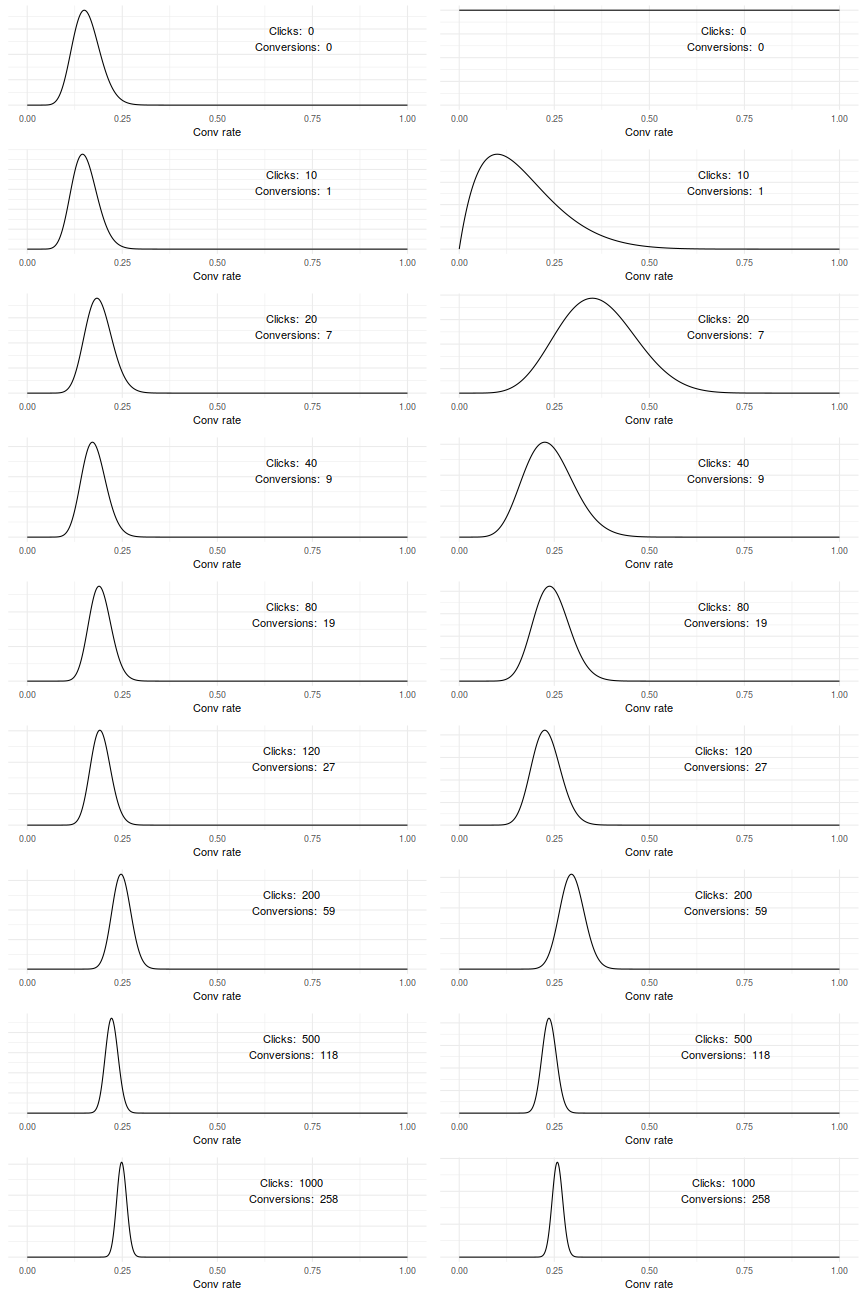The end estimate, when there is lots of data, is practically identical but earlier estimates when there is not so much data is influenced more by the prior information. As we gather more real keyword data the influence of the prior on the posterior distribution is lower and lower. Great! Now we are in a situation where: 1. We get an estimate of the distribution of the conversion rate for each keyword 2. We don’t have to worry about specifying that pesky λ But we do have to worry about specifying the prior distribution. How do we know that giving every keyword 15 conversions and 100 clicks to start with is the right way of doing it? What data do we have to back up this assumption? And this assumption does make some difference. For example, here is the same plot as above but with a much narrower prior distribution (based on 1500 conversions and 10000 clicks):  makeplotPrior <- function(clicks,conversions) { df <- betadata(clicks+10000,conversions+1500) maxdent <- max(df$d)
ggplot(df,aes(x=xs,y=d)) +
geom_line() +
theme_minimal() +
xlab("Conv rate") +
ylab(NULL) +
scale_y_continuous(labels=NULL) +
expand_limits(y=0) +
annotate("text", label=paste("Clicks: ",clicks,"\nConversions: ", conversions,sep=" "), x=0.7, y=maxdent*0.7)
}
multiplot(makeplotPrior(0,0),
makeplotPrior(10,1),
makeplotPrior(20,7),
makeplotPrior(40,9),
makeplotPrior(80,19),
makeplotPrior(120,27),
makeplotPrior(200,59),
makeplotPrior(500,118),
makeplotPrior(1000,258),
makeplot(0,0),
makeplot(10,1),
makeplot(20,7),
makeplot(40,9),
makeplot(80,19),
makeplot(120,27),
makeplot(200,59),
makeplot(500,118),
makeplot(1000,258), cols=2)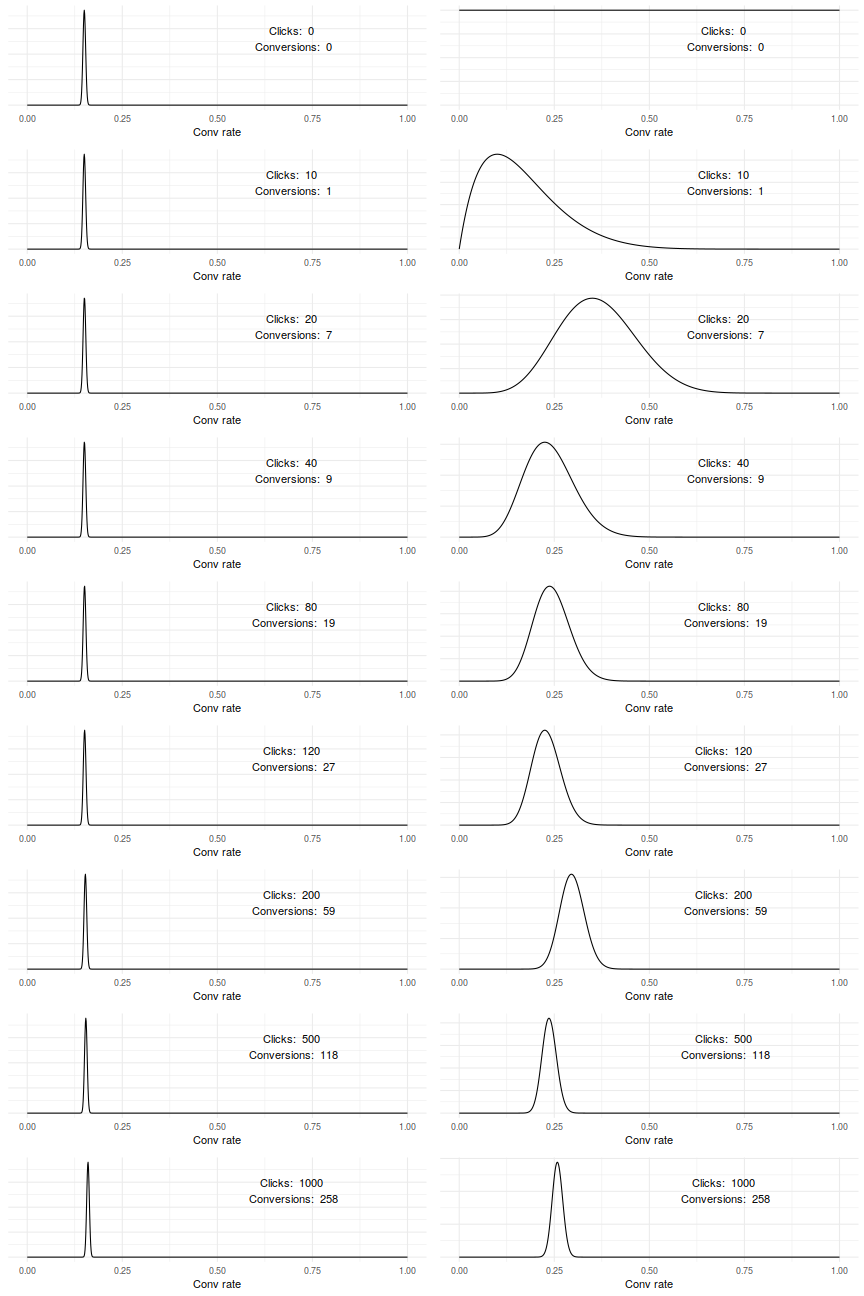After 1000 clicks and 258 conversions we should be fairly sure that the true conversion rate is about 25% but our distribution of expected conversion rate does not reflect this at all!

How we specify our prior information does effect the predictions our model makes. So we don’t want to do this in an arbitrary way.

It is actually perfectly possible to do this in an arbitrary way. I just think it is inelegant to do so.

## Multi-level Models

To start with our “trick” was to stop considering the conversion rate as a fixed quantity and to start thinking of it as a random variable with a distribution.

We can do the same thing with our prior information - rather than thinking of the beta distribution parameters as fixed quantities we can also think of them as a random variable.

This is a multi-level model because we have parameters (our binomial conversion rates) and hyper-parameters which control the prior distribution for the parameters.

We could keep going with this and have hyper-hyper-parameters and hyper-hyper-hyper-parameters and so on, but for now I’m going to stick with the simpler model.

### Model fitting

The standard way of fitting these models is to use Monte Carlo methods. I’ll talk a bit about this here, but I won’t go into enough depth to do it justice.

Given a model and some data it is quite easy to say "what is the probability that we observe this data from this model. Here is an example from the Normal distribution:

observeddata = [3, 4, 6]

Then the probability a single observation came from 𝒩(4, 1) (normal distribution with mean 4 and variance 1) is the value of the probability density function for 𝒩(4, 1) at that observation.

Let F(x) be the probability density function. Then the probability of seeing the observed data under this model is:

P(observeddata|model) = F(3)F(4)F(6)

P(observeddata|model) = 0.242 * 0.399 * 0.054

P(observeddata|model) = 0.005

The point is that calculating the likelihood of some observations is normally pretty easy.

Bayes’ Theorem tells us that the likelihood of a model given the data is proportional to the likelihood of the data given the model. This tells us that the most likely model is the one that maximises the likelihood of the observations.

This can be used to get a point estimate for the model parameters. But we don’t want a point estimate, we want to know how our parameter estimates are distributed.

This is where the Monte Carlo bit comes in.

Every model has a parameter space where every point in the space represents a combination of values for the parameters. In a model with two unconstrained, real number parameters the parameter space is the 2D plane with the value on the x-axis representing one parameter and the value on the y-axis representing the other parameter.

MCMC sampling algorithms describe different ways of moving around the parameter space. The cool bit is that when you look at a list of the points visited it is as if they are all sampled from the distributions we want to estimate. We can plot histograms and find quartiles etc. and generally get all the benefits we need.

The rules are quite simple:

1. Start at a point pi in the parameter space
2. Generate a new candidate point pcandidate
3. If Likelihood(pcandidate) ≥ Likelihood(pi) then move to pcandidate (set pi + 1 = pcandidate)
4. Otherwise move to pcandidate with probability $\frac{Likelihood(p_{candidate})}{Likelihood(p_i)}$
5. If no move is made then pi + 1 = pi

The sampler spends more time in areas of high likelihood and less time in areas of low likelihood.

The tricky part with this is making sure that the sampler explores the whole parameter space and doesn’t get stuck in a local maxima. But if the sampler tries to move too far or too aggressively the candidate points are more likely to be rejected leaving the sampler stuck in one place.

In other words, sampling from non-trivial parameter spaces is hard and naive approaches sometimes have nasty pitfalls. Luckily there are open source samplers we can use instead.

I’m a fan of Stan, mainly because it has good marketing , an R interface and a nice way to explore models.

It is also supposed to be fast (sampler is converted to C++ and compiled before running) and have one of the best sampling algorithms (NUTS) which all sounds cool but I haven’t played around with the other options enough to comment.

Stan requires you to write your model in it’s own domain specific language. This could be considered a strength or a weakness. An example is given below:

    library(rstan)
rstan_options(auto_write = TRUE)
options(mc.cores = parallel::detectCores())

# normally this would go in a separate file
# but embedding it here is easier
stanmodel <- "data {
int<lower=0> J; // number of keywords
int<lower=0> conv[J]; // number of conv for kwd j
int<lower=0> click[J]; // number of clicks for j
}
parameters {
real<lower=0,upper=1> theta[J]; // true conv rate for j
real<lower=0> alpha; // prior conv count
real<lower=0> beta; // prior fail count
}
model {
// distribution parameters for the hyperpriors
// are chosen arbitrarily (this matters less here)
alpha ~ exponential(0.1); // hyperprior
beta ~ exponential(0.1); // hyperprior
theta ~ beta(alpha+1,beta+1); // prior
conv ~ binomial(click,theta); // likelihood
}"

keyword_data <- list(J = 4,
conv = c(0,1,5,10),
click = c(0,10,50,100)
)

fit <- stan(model_code=stanmodel,
data=keyword_data,
iter=1000,
chains=4)

We can plot data from the fit object to see what our estimated distributions look like:

    library(rstan) # needed because above block is cached
    # fit has a plot method so we could just do
# > plot(fit)
# but this way makes things slightly clearer I think

samples <- extract(fit)
samples <- samples$theta samples <- as.data.frame(samples) names(samples) <- c("Keyword1","Keyword2","Keyword3","Keyword4") plotDensity <- function(colnum) { columnname <- paste("Keyword",colnum,sep="") clicks <- keyword_data$click[colnum]
conv <- keyword_data$conv[colnum] ggplot(samples,aes_string(x=columnname)) + geom_density() + theme_minimal() + xlab("Conv Rate") + xlim(0,1) + ylab(NULL) + scale_y_continuous(labels=NULL) + expand_limits(y=0) + annotate("text", label=paste("Clicks: ",clicks,"\nConversions: ", conv,sep=" "), x=0.7, y=3) } multiplot(plotDensity(1), plotDensity(2), plotDensity(3), plotDensity(4), cols=1)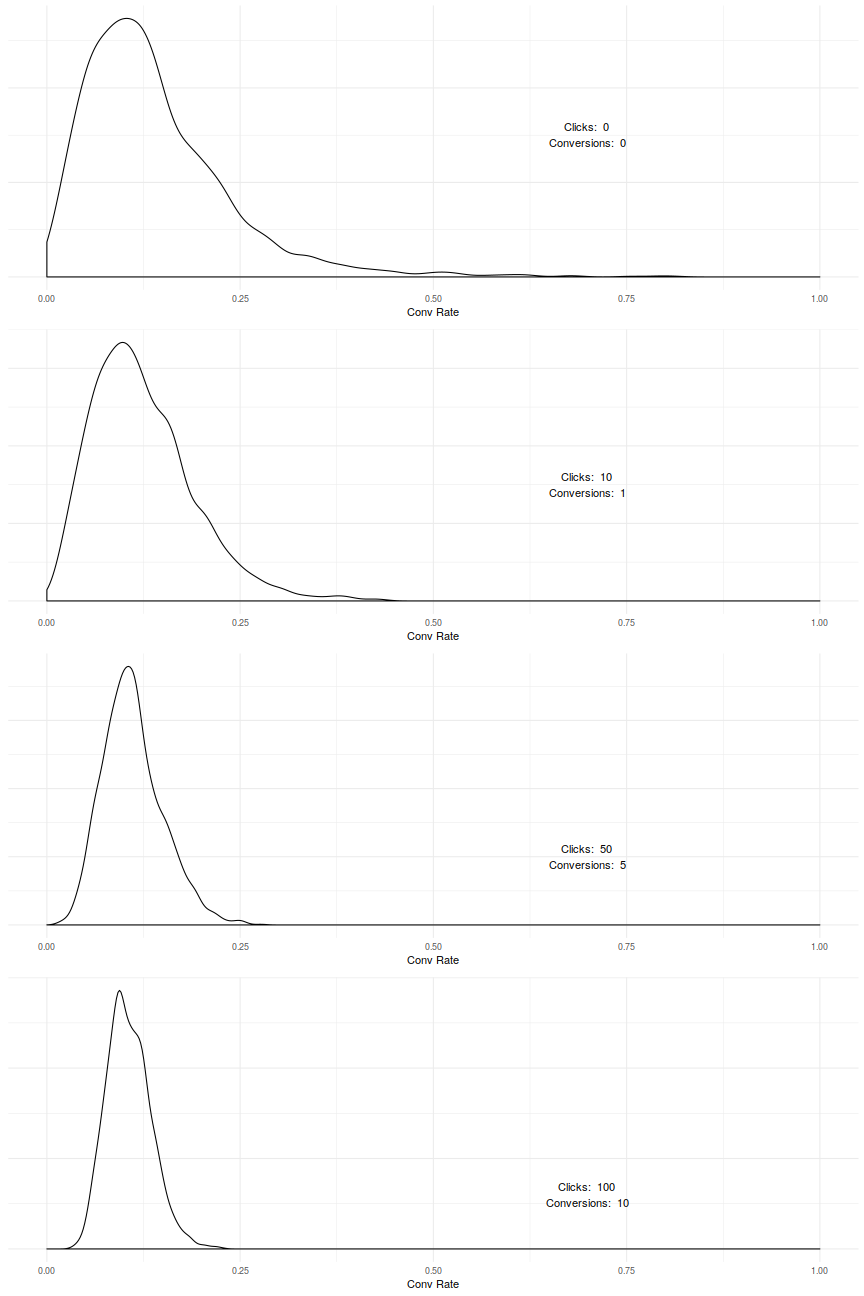As we have more keyword data our estimates narrow, as we would expect. In the example keyword_data, all keywords have the same conversion rate of 10%. This means we can see how the distributions converge as more information is known, but it is important to remember that the information we have on all the other keywords changes our estimate of the distribution for a single keyword. Here is an example to demonstrate:  keyword_data <- list(J = 4, conv = c(0,1,20,10), click = c(0,10,50,100) )  fit2 <- stan(model_code=stanmodel, data=keyword_data, iter=1000, chains=4, verbose=FALSE)  samples <- extract(fit2) samples <- samples$theta
samples <- as.data.frame(samples)
names(samples) <- c("Keyword1","Keyword2","Keyword3","Keyword4")
multiplot(plotDensity(1),
plotDensity(2),
plotDensity(3),
plotDensity(4),
cols=1)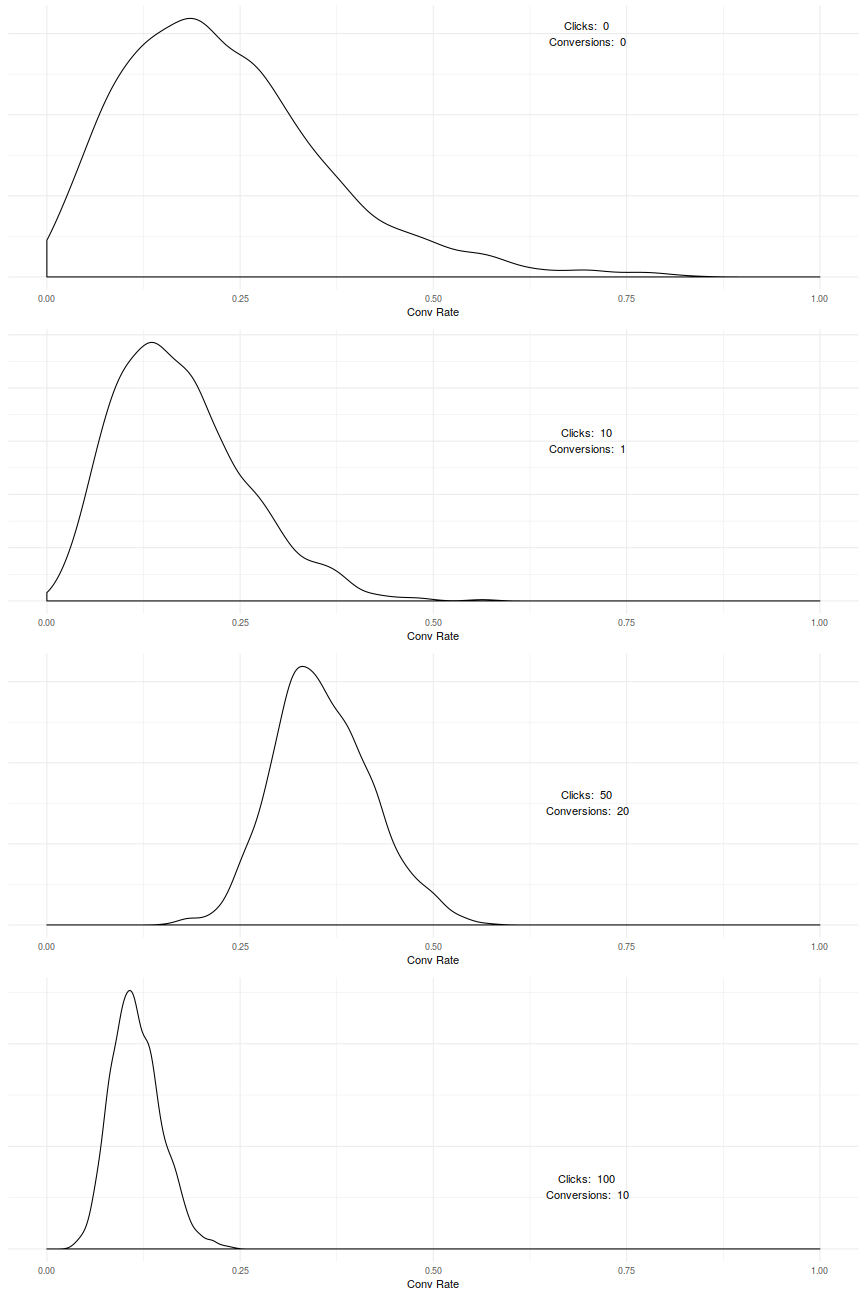Having a keyword with a 40% conversion rate really changes the model results for keywords that don’t have much data.

This is what I want to happen! Remember our modelling intuition here is that “this keyword is kind of like all the other keywords in the account”. So we’d expect the model to be less certain about the conversion rate when it sees that some keywords have a 10% conversion rate and some keywords have a 20% conversion rate.

The way the model is fitted takes into account that when we have a lot of keyword level data we don’t care so much about the account level average. And that when we don’t have much keyword level data the account level data (and the variability between keywords) is more important.

But what if we want to make different assumptions into a more complicated model?

### More complicated models

The latest model is based on the intuition that “this keyword is a bit like all the other keywords in the account”. This is true to a certain extent but PPCers tend to hate aggregate assumptions like this because a lot of the job is based around “de-aggregating” along biddable dimensions and exploiting the efficiencies that result.

So I think we can do better with more specific assumptions.

Unfortunately, at this point (or maybe slightly earlier :-) ), we reach the end of my competence. There are some bits in what follows that kind of work but a lot of the elegance in what came before is lost.

A loss of elegance is necessary at some point because the real world is not an elegant place. But right now I feel that the elegance is lost because I am not clever enough to maintain it rather than because the real world is imposing inelegant things upon me.

So expect the following to be a bit less coherent than what has previously gone before.

#### Stan and Ragged Arrays

Trying to build a model around the idea “this keyword is a bit like other keywords in the same subgroup of the account” sets us up for the obvious approach of partitioning the account into these subsections and then running our model over each subsection separately.

Yuck! This method ignores any information that the account as a whole can tell us about any particular subsection. It is kind of equivalent to only looking at data for a single keyword when setting a bid. What happens when a subsection has very little data?

One way to share some information between subsections is to have a beta distribution for each subsection where the beta distribution hyper-parameters are sampled from some shared distribution. i.e. each subsection has it’s own beta distribution but these beta distributions are, in some way, similar.

The stan code for this is a bit ugly:

      data {
int J; // number of keywords
int G; // number of groups
int s[G]; // number of kwds in each group
int conv[J]; // number of conv for kwd j
int click[J]; // number of clicks for j
}
parameters {
real theta[J]; // true conv rate for j
real alpha[G]; // prior conv count (1 for each group)
real beta[G]; // prior fail count (1 for each group)
real eta1; // hyper-prior for alpha
real eta2; // hyper-prior for beta
}
model {
int pos;
pos <- 1; // an iterator for how far through the array we are
for (g in 1:G) { // for each group
alpha[g] ~ exponential(eta1);
beta[g] ~ exponential(eta2);
segment(theta, pos, s[g]) ~ beta(alpha[g]+1,beta[g]+1);
segment(conv, pos, s[g]) ~ binomial(segment(click, pos, s[g]), segment(theta, pos, s[g]));
pos <- pos + s[g];
}
}


Running this model through Stan spits out a load of warning messages that I don’t understand yet. But the fitting looks reasonably sound.

    # model as above
stanmodel2 <- [1380 chars quoted with '"']

keyword_data <- list( J = 6,
G = 2,
s = c(5,1),
conv = c(10,10,10,1,0,0),
click = c(100,100,100,10,0,0)
)

fit3 <- stan(model_code=stanmodel2,
data=keyword_data,
iter=1000,
chains=4,
verbose=FALSE)
    samples <- extract(fit3)
samples <- samples\$theta
samples <- as.data.frame(samples)
names(samples) <- c("Keyword1","Keyword2","Keyword3","Keyword4","Keyword5","Keyword6")
multiplot(plotDensity(1),
plotDensity(4),
plotDensity(5),
plotDensity(6),
cols=1)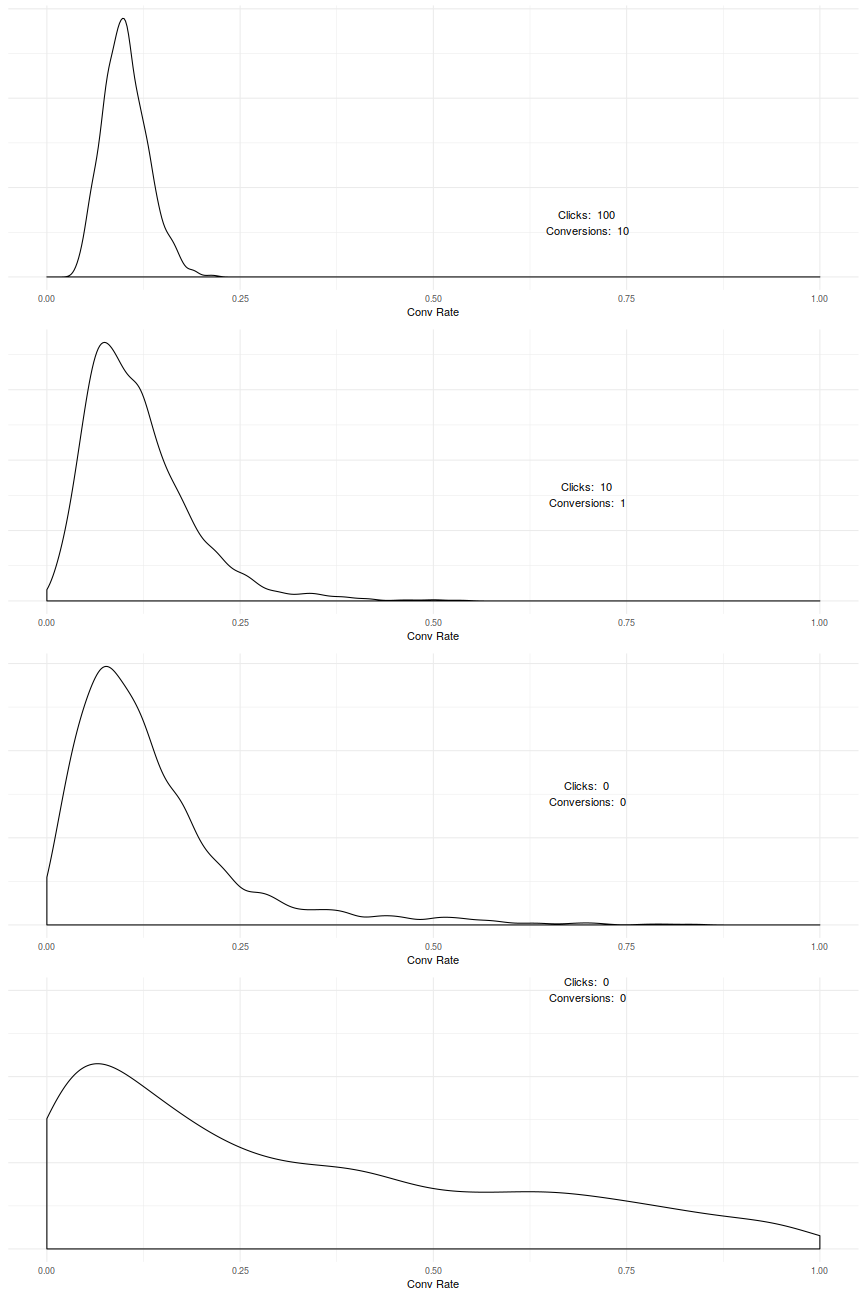Note how in the final two plots we have the same keyword level data but the distributions look very different because the first keyword is in the first group and the second keyword is in the second group. The second group also has no performance data so any information in the density comes from the overall account performance [IS THIS TRUE?].

Unfortunately fitting this kind of model on real world data seems to take a very long time. Actually, fitting our earlier model is also pretty slow, but things are worse here. This is for two reasons:

1. The likelihood function takes longer to calculate when running the sampler
2. The rejection rate of the sampler is higher

The second is an inevitable consequence of having more parameters to estimate. Stan uses what is currently considered to be the most advanced/best sampler for general use. There may be some feature of this problem that makes another sampling algorithm more appropriate but I don’t know what this is.

The first is partly because Stan is unable to “vectorise” the likelihood calculation. In the earlier example there was no need for for loops which allows Stan to make a few optimisations in how the likelihood is calculated. When we move to the second model groups may be different sizes and the only way Stan supports these “ragged arrays” is with weird pos and segment.

I think a faster likelihood function is probably possible in this case. I’m playing with doing this in Haskell for three reasons:

1. I like Haskell - and at this stage this is definitely a “fun” project
2. I can use file-embed to embed the data directly in the executable at compile time. This gives a quicker likelihood function on the simple things I’ve tested
3. There is excellent support for automatic differentiation which is a pretty important part of the more complicated sampling algorithms.

Right now, I have code that can fit a normal distribution fairly quickly. But everything can do that and it isn’t that hard. It gets more difficult with higher dimensionality and tightly constrained parameters.

That is about as far as I’ve got. I’m sad this is ending with a whimper rather than a bang but this is where I am right now.